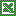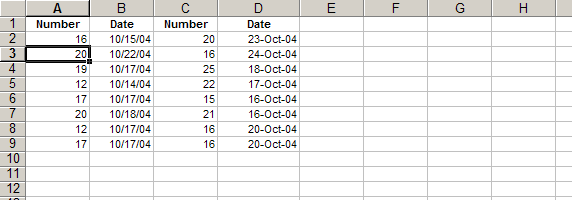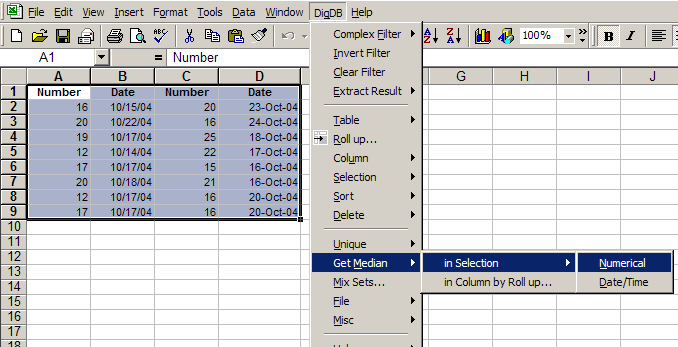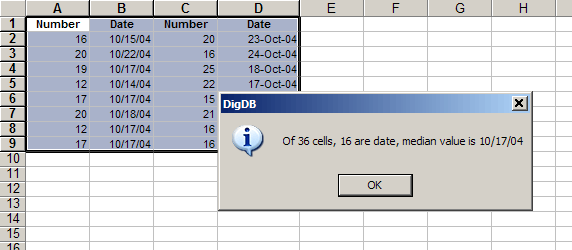DigDBAdd-in Tools for Excel Elites
Who else wants to do a 10-hour Excel analysis in 15 minutes? (more ...)

Features | Testimonial | Download free 15-day trial | Purchase | FAQ | Contact

### Calculate Median in Selected Range

Select any range or ranges, quickly calculate the median number value or date value. Excel's median formula will fail when the range contains non-numeric values. And Excel's median formula does not differentiate between date and number values.

The Median is the number in the middle of a set of numbers; that is, half the numbers have values that are greater than the median, and half have values that are less. If there is an even number of numbers in the set, then MEDIAN calculates the average of the two numbers in the middle. For example:

• MEDIAN of the set of numbers (1, 2, 3, 4, 5) equals 3
• MEDIAN of the set of numbers (1, 2, 3, 4, 5, 6) equals 3.5, the average of 3 and 4Practice file - median-in-range-demo.xls (16k)

1. Select the range(s) first, (use Ctrl+select for multiple selections), then invoke 'DigDB->Get Median->in Selection->Numerical...'If you choose Get Median->Numberical, only the number values will be included in the median calculation.If you choose Get Median->Date/Time, only the Date/Time values will be included in the median calculation.If you can trying to get median value in a pivot table kind of way, you should use the "DigDB->Get Median->in Column by Roll-up...". This function is the same as the Roll up with median demo.

### Need more?

• Browse the complete list of DigDB's powerful features listed on the front page.
• If you see errors or things not working, check out our error reference page.

DigDBAdd-in Tools for Excel Elites